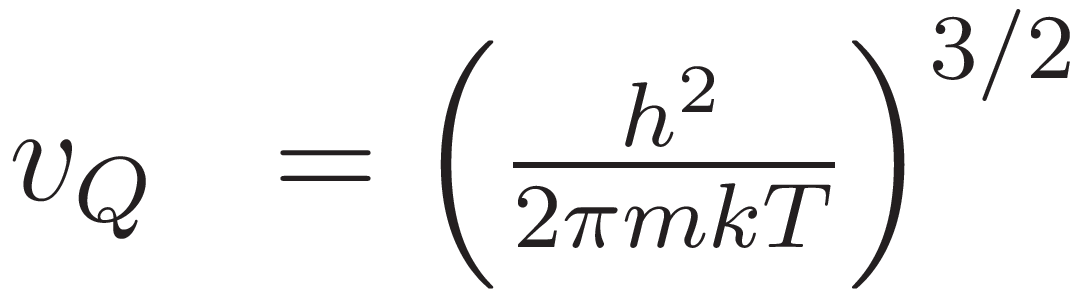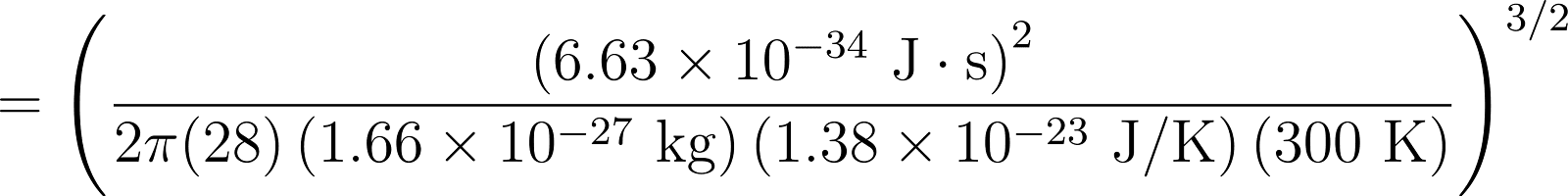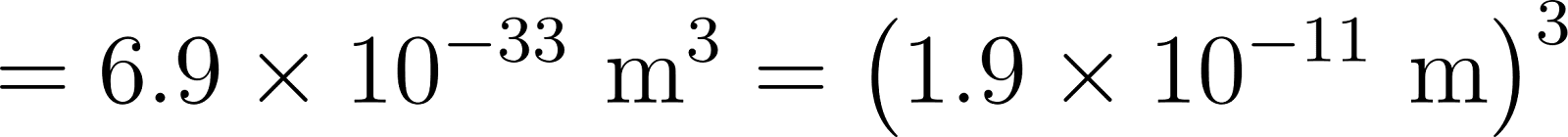×
Get Full Access to An Introduction To Thermal Physics - 1 Edition - Chapter 7 - Problem 9p
Get Full Access to An Introduction To Thermal Physics - 1 Edition - Chapter 7 - Problem 9p

×

# Compute the quantum volume for an N2 molecule at roomISBN: 9780201380279 40

## Solution for problem 9P Chapter 7

An Introduction to Thermal Physics | 1st Edition

• Textbook Solutions
• 2901 Step-by-step solutions solved by professors and subject experts
• Get 24/7 help from StudySoup virtual teaching assistantsAn Introduction to Thermal Physics | 1st Edition

4 5 1 237 Reviews
22
1
Problem 9P

Problem 9P

Compute the quantum volume for an N2 molecule at room temperature, and argue that a gas of such molecules at atmospheric pressure can be treated using Boltzmann statistics. At about what temperature would quantum statistics become relevant for this system (keeping the density constant and pretending sthat the gas does not liquefy)?

Step-by-Step Solution:

Step 1 of 3

Mass of an nitrogen moleculeSo its quantum volume at room temperatureStep 2 of 3

Step 3 of 3

##### ISBN: 9780201380279

Unlock Textbook Solution